# Math in Focus Grade 3 Chapter 6 Practice 3 Answer Key Multiply by 7

Go through the Math in Focus Grade 3 Workbook Answer Key Chapter 6 Practice 3 Multiply by 7 to finish your assignments.

## Math in Focus Grade 3 Chapter 6 Practice 3 Answer Key Multiply by 7

Example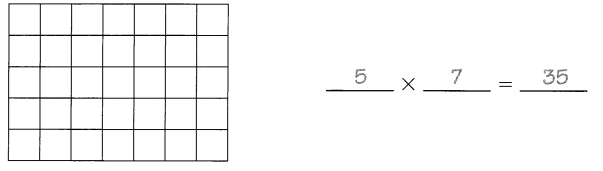Question 1.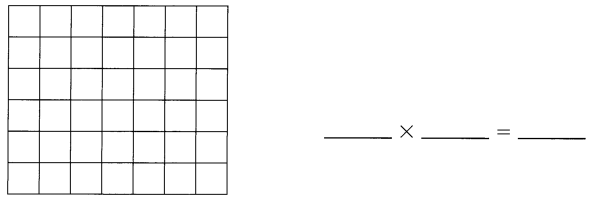6×7 = 42.

Explanation:
In the above image, we can see that the array of 6×7 which is 42.

Question 2.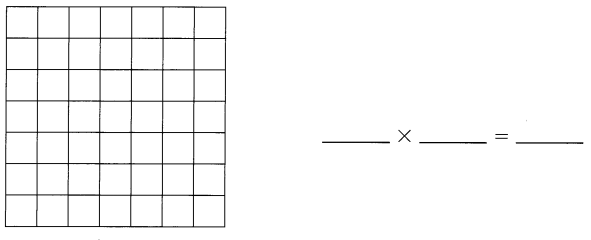7×7 = 49.

Explanation:
In the above image, we can see that the array of 7×7 which is 49.

Fill in the missing numbers.

Question 3.
6 sevens = 6 × ___
6 sevens = 6 × 7 = 42.

Explanation:
Given that 6 sevens which is 6 × 7 = 42.

Question 4.
9 × 7 = ___ sevens
9 × 7 = 9 sevens.

Explanation:
Given that 9 × 7 which have 9 sevens which is 63.

Question 5.
5 + 5 + 5 + 5 + 5 + 5 + 5 = 7 × ___
5 + 5 + 5 + 5 + 5 + 5 + 5 = 7 × 5 = 35.

Explanation:
Given that 5 + 5 + 5 + 5 + 5 + 5 + 5 which can be also 7×5 = 35.

Question 6.
10 × 7 = 7 × ___
10 × 7 = 7 × 10 =70.

Explanation:
Here, by using commutative property we can write 10 × 7 = 7 × 10 = 70.

Multiply. Use multiplication facts you know to and other multiplication facts.

Question 9.
7 × 4 = 5 groups of 4 + ___ groups of 4
= ____ + ____
= ____
7 × 4 = 28.

Explanation:
Given that 7 × 4 which is
= 5 groups of 4 + 2 groups of 4
= 20+8
= 28.

Question 10.
5 × 7 = ___
7 × 7 = 5 groups of 7 + __ groups of 7
= ____ + ____
= _____
5 × 7 = 35,
7 × 7 = 49.

Explantion:
Given that 5 × 7 which is 35,
5 × 7 = 35
7 × 7 = 5 groups of 7 + 2 groups of 7
= 35+14
= 49.

Question 11.
10 × 7 = ____
9 × 7 = 10 groups of 7 – ___ groups of 7
= ____ + ____
= ___

10 × 7 = 70.
9 × 7 = 63.

Explanation:
Given that 10 × 7 which is 70,
9 × 7 = 10 groups of 7 – 1 groups of 7
= 70 + 7
= 63.

Multiply and match.

Question 12.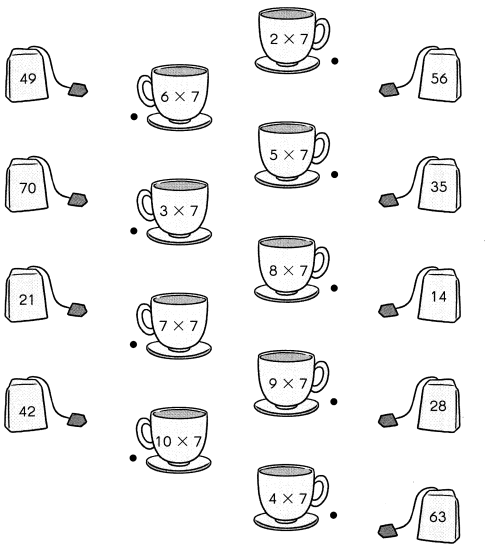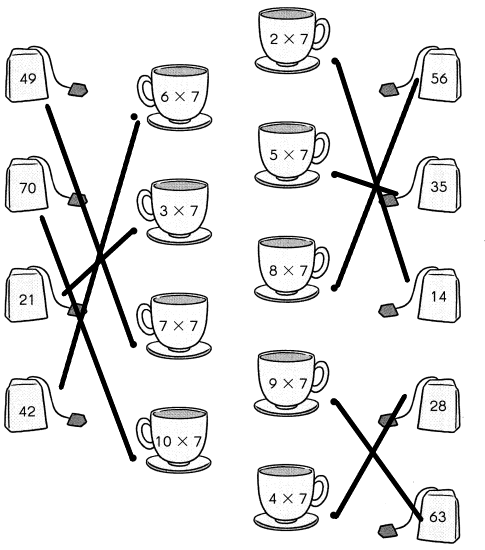Solve.

Question 13.
Mrs. Thompson buys 2 books. Each book costs $7. How much does Mrs. Thompson pay in all? 2 ×$7 = $___ Mrs. Thompson pays$__________ in all.
Mrs. Thompson pays $14 in all. Explanation: Given that Mrs. Thompson bought 2 books and each book costs$7. So the total cost of Mrs. Thompson pay in all is 2 × $7 which is$14.

Question 14.
A box contains 7 crayons.
Alex packs 10 such boxes into his bog.
How many crayons does Alex have in all?
10 × 7 = ______
Alex has ___ crayons in all.
Alex has 70 crayons in all.

Explanation:
Given that a box contains 7 crayons and Alex packs 10 such boxes into his box. So the number of crayons does Alex have in all is 7 × 10 is 70 crayons.

Question 15.
Mr. Dean gives each student 7 okras in art class.
How many okras does he give 4 students?
4 × 7 = ___
He gives 4 students ___ okras.